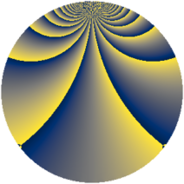# Properties

 Label 1425.2.bpLevel $1425$ Weight $2$ Character orbit 1425.bp Rep. character $\chi_{1425}(37,\cdot)$ Character field $\Q(\zeta_{20})$ Dimension $800$ Sturm bound $400$

# Related objects

## Defining parameters

 Level: $$N$$ $$=$$ $$1425 = 3 \cdot 5^{2} \cdot 19$$ Weight: $$k$$ $$=$$ $$2$$ Character orbit: $$[\chi]$$ $$=$$ 1425.bp (of order $$20$$ and degree $$8$$) Character conductor: $$\operatorname{cond}(\chi)$$ $$=$$ $$475$$ Character field: $$\Q(\zeta_{20})$$ Sturm bound: $$400$$

## Dimensions

The following table gives the dimensions of various subspaces of $$M_{2}(1425, [\chi])$$.

Total New Old
Modular forms 1632 800 832
Cusp forms 1568 800 768
Eisenstein series 64 0 64

## Trace form

 $$800q + 4q^{5} - 4q^{7} + O(q^{10})$$ $$800q + 4q^{5} - 4q^{7} + 200q^{16} + 16q^{17} - 20q^{19} + 40q^{20} - 48q^{23} - 20q^{25} + 8q^{28} + 64q^{30} - 64q^{35} - 200q^{36} - 36q^{38} - 124q^{43} + 280q^{44} + 172q^{47} - 80q^{55} + 176q^{58} - 192q^{62} + 4q^{63} - 120q^{64} + 40q^{68} + 4q^{73} + 68q^{77} - 168q^{80} + 200q^{81} + 72q^{82} - 48q^{83} + 88q^{85} - 128q^{87} + 256q^{92} + 192q^{93} - 196q^{95} + 40q^{96} + O(q^{100})$$

## Decomposition of $$S_{2}^{\mathrm{new}}(1425, [\chi])$$ into newform subspaces

The newforms in this space have not yet been added to the LMFDB.

## Decomposition of $$S_{2}^{\mathrm{old}}(1425, [\chi])$$ into lower level spaces

$$S_{2}^{\mathrm{old}}(1425, [\chi]) \cong$$ $$S_{2}^{\mathrm{new}}(475, [\chi])$$$$^{\oplus 2}$$